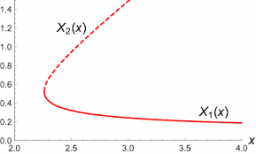# Calculate 70804

The garden has the shape of a right triangle and is fenced with a fence length of 364m. The shorter slope of the triangle is 26 m long. Calculate the area of ​​this garden.

A =  2184 m2

### Step-by-step explanation:Did you find an error or inaccuracy? Feel free to write us. Thank you!

Tips for related online calculators
Are you looking for help with calculating roots of a quadratic equation?
Need help calculating sum, simplifying, or multiplying fractions? Try our fraction calculator.
Do you have a linear equation or system of equations and looking for its solution? Or do you have a quadratic equation?